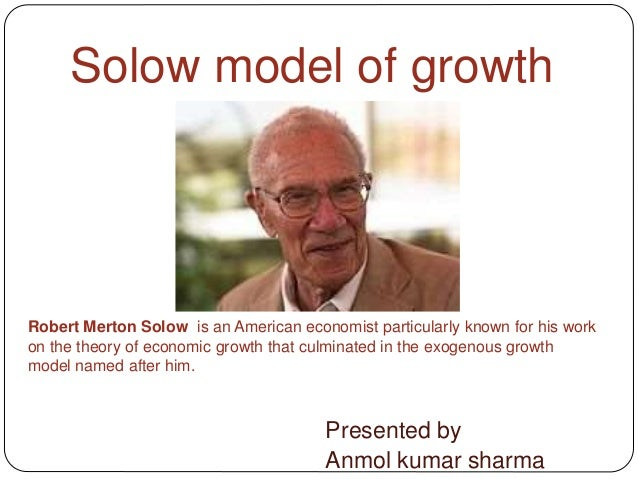Successfully reported this slideshow.
We use your LinkedIn profile and activity data to personalize ads and to show you more relevant ads. You can change your ad preferences anytime.Upcoming SlideShare
Loading in …5
×

# Solow model of growth

Prof R.M solow gave this model as an alternative to Harrod domar model of growth. It is a basic neo-classical model.

• Full Name
Comment goes here.

Are you sure you want to Yes No
Your message goes here• Be the first to comment

### Solow model of growth

1. 1. Solow model of growth Presented by Anmol kumar sharma Robert Merton Solow is an American economist particularly known for his work on the theory of economic growth that culminated in the exogenous growth model named after him.
2. 2. Prof. R.M.Solow builds his model of economic growth as an alternative to the Harrod-Domar model of growth without its crucial assumption of fixed proportion in production. He brings a continuous production function linking output to input of Labor and capital which are substitutable Solow model of growth
3. 3. Assumptions  One composite commodity is produced  Output is net output after depreciation of capital  Labor and capital are substitutable  Prices and wages are flexible  There is full employment of labor  There is constant returns to scale  There is neutral technical progress  The saving ratio is constant  The two factors of production land and capital are paid according to their marginal physical productivities
4. 4. Basic idea  In Solow model there would be tendency for K/L ( capital labor ratio) to adjust itself through time in the direction of equilibrium ratio. If the initial ratio of capital to labor is more capital and output would grow more slowly than labor force and vice versa. Solow’s analysis is convergent to equilibrium path to start with any capital labor ratio.
5. 5. Explanation  Solow takes output as a whole the only commodity in the economy.  Annual rate of production is Y(t) part of it is consumed and rest is saved and invested.  That which is saved is a constant s, and rate of saving is s Y(t)  K(t) is the stock of capital and net investment or rate of increase of this stock of capital is K
6. 6. Equations . Basic equation is K=sY …(1)equation  Since output is produced with capital and labor, technological possibilities are represented by the production function Y=F(K,L) that shows constant returns to scale..(2)equation Inserting equation 2 in 1 we get K=sF(K,L) (3)equation
7. 7. Continued..  In equation 3 i.e. K=sF(K,L) L represents total employment since population is growing exogenously, the labor force increases at a constant relative rate n thus fourth equation is L(t) = Loe nt Solow regards n as Harrod natural rate of growth in the absence of technological change and L(t) as the available supply of labor at the rate t
8. 8. Final equation  By inserting equation 4 in 3 we get K =sF(K, Loe nt) He regards this basic equation of as determining the time path of capital accumulation K that must be followed if all available labor is to be fully employed.
9. 9. sF(r,1) nr r o Fig 1  Equation r=sF(r,1)-nr  here r is the K/L ratio of capital to labor  N is relative change of labor force L/L  The function r=sF(r,1) represents output per worker as a function of capital per worker. It is total product curve as varying amounts of capital are employed with one unit of labor  In fig 1 ray through origin is the function nr the other curve is funtion sF(r,1) which shows diminishing mu of capital. At the point of intersection of the two curves nr sF(r,1) and r=0  Here K/L is a constant and captial stock must expand at same rate of labor force Growth pattern I
10. 10. r1 r2 r3 sF(r,1) nr o r Growth pattern II In Fig 2 the productivity curve sF(r,1) intersects the curve nr at three points r1, r2, r3 . Here r1 and r3 are stable equilibrium positions because the total productivity curve is above nr but at r2 it is below nr and it is unstable
11. 11. Growth pattern III In Fiq 3 the ray nr depicts equilibrium path where warranted and natural growth rates are equal the curve sF’(r,1) which is above nr represents a highly productive system in which capital and income increase more rapidly than the labor supply thn this system, which is of perpetual full employment, income and saving increase so much that the capital labor ratio in crease limitlessly on the other hand curve S2F”(r,1) depicts highly unproductive system in which the full employment path leads to ever diminishing per capital income S1 F(r,1) o S2 F”(r,1) nr r
12. 12. summary  Professor Solow conclusion of his model is “ when production takes place under the usual neo-classical conditions of variable proportions and constant returns to scale, no simple opposition between natural and warranted growth rates is possible. There may not be any knife edge. The system can adjust to any given rate of growth of the labor force, and eventually approach a state of steady proportional expansion i.e. ∆K/K = ∆L/L = ∆Y/Y
13. 13. Critical appraisal  The Solow model is a major improvement over the Harod-Domar model as in H-D model there is a knife edge and is any of the used parameters change a bit consequences would be chronic inflation or unemployment. While assumption of substitutability between labor and capital gives the growth process an adjustability and problem of Knife edge doesn’t arise.
14. 14. Note: This slide is to make Solow model easy for beginners and therefore suitable adjustments have been made to simplify the model. Thank you